# Diffusion Schrodinger Bridge

Published:

Diffusion Schrödinger Bridge with Applications to Score-Based Generative Modeling.

## Contributors

Valentin De Bortoli, James Thornton, Jeremy Heng, Arnaud Doucet

## What is a Schrödinger bridge?

The Schrödinger Bridge (SB) problem is a classical problem appearing in applied mathematics, optimal control and probability; see [1, 2, 3]. In the discrete-time setting, it takes the following (dynamic) form. Consider as reference density p(x0:N) describing the process adding noise to the data. We aim to find p*(x0:N) such that p*(x0) = pdata(x0) and p*(xN) = pprior(xN) and minimize the Kullback-Leibler divergence between p* and p. In this work we introduce Diffusion Schrodinger Bridge (DSB), a new algorithm which uses score-matching approaches  to approximate the Iterative Proportional Fitting algorithm, an iterative method to find the solutions of the SB problem. DSB can be seen as a refinement of existing score-based generative modeling methods [5, 6].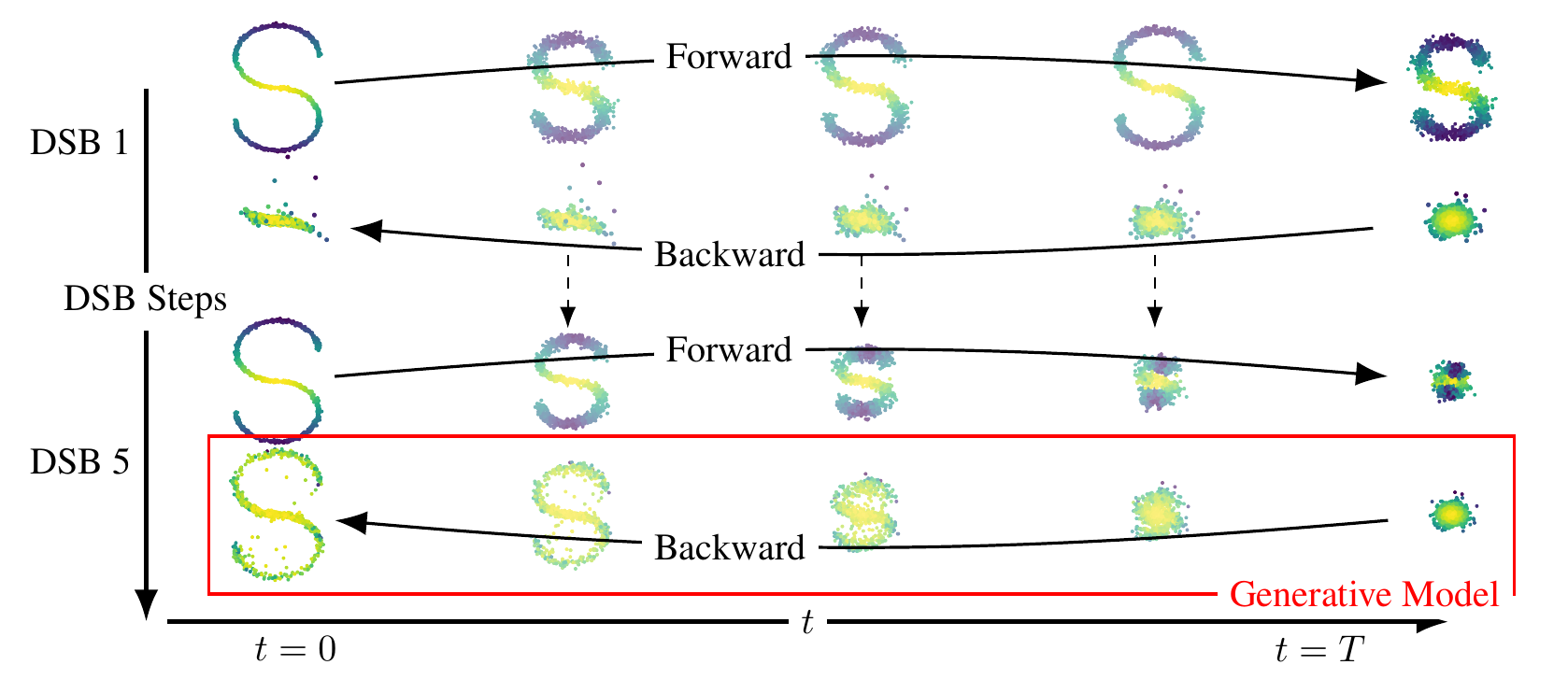## Two dimensional examples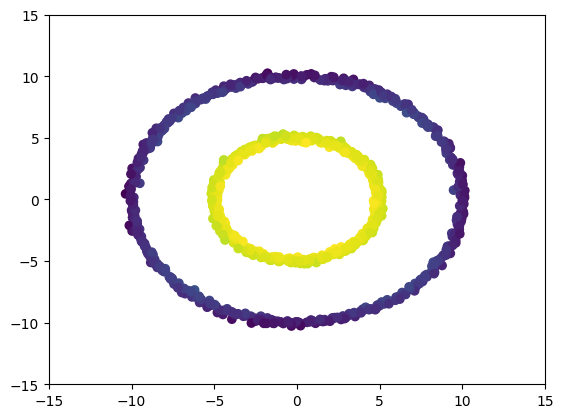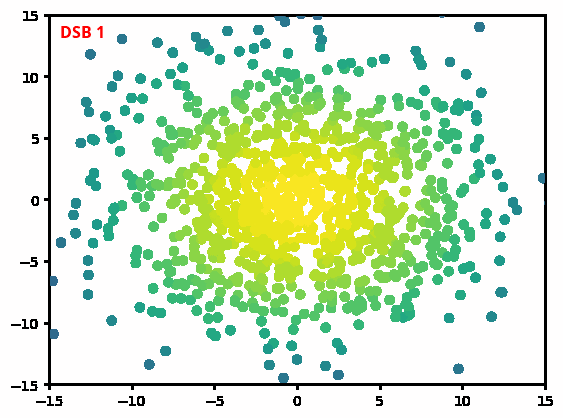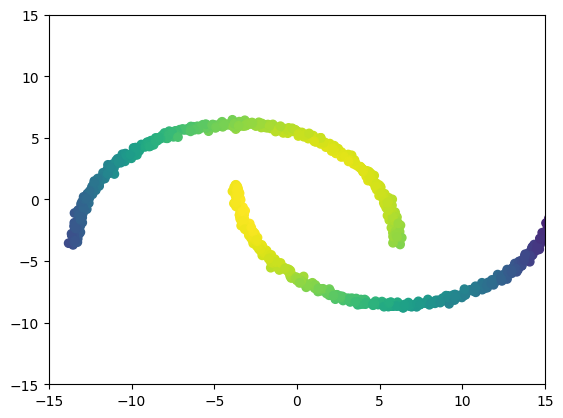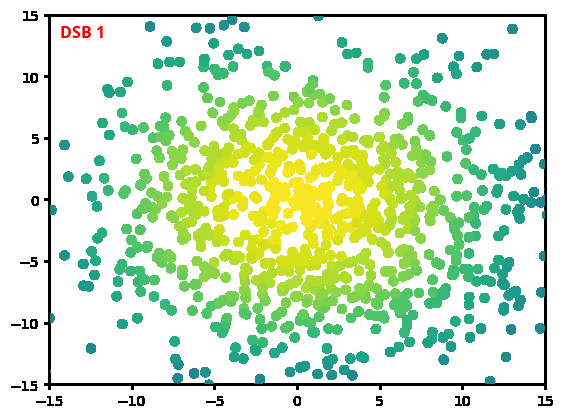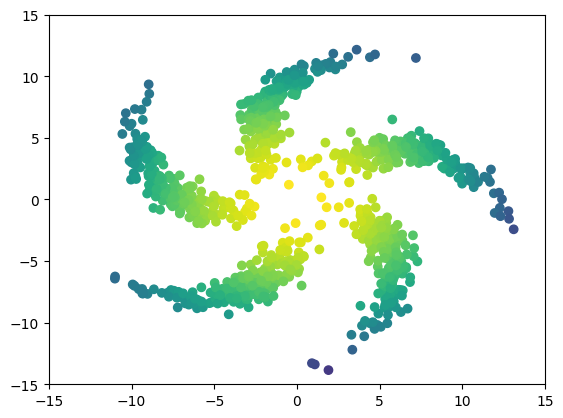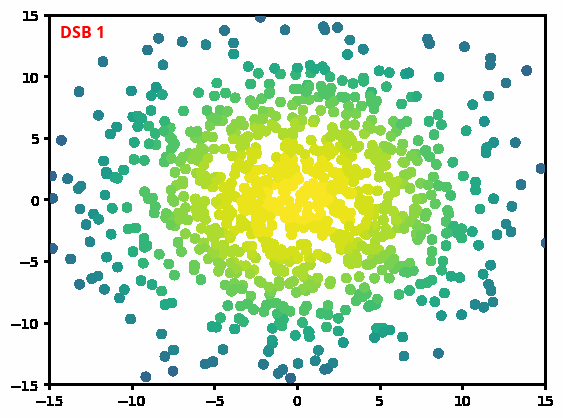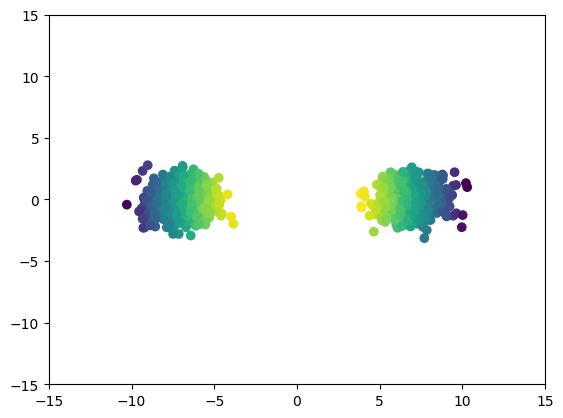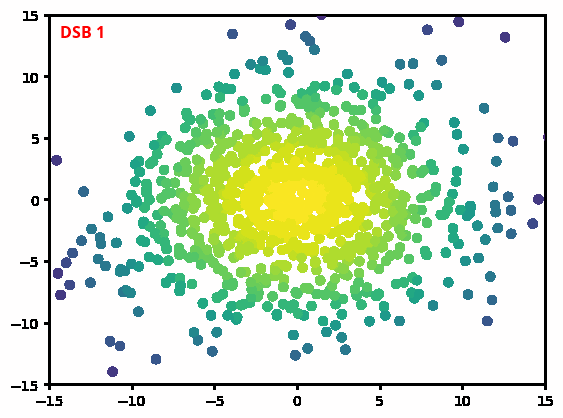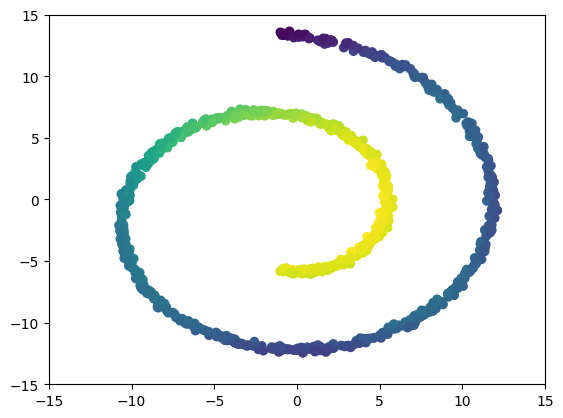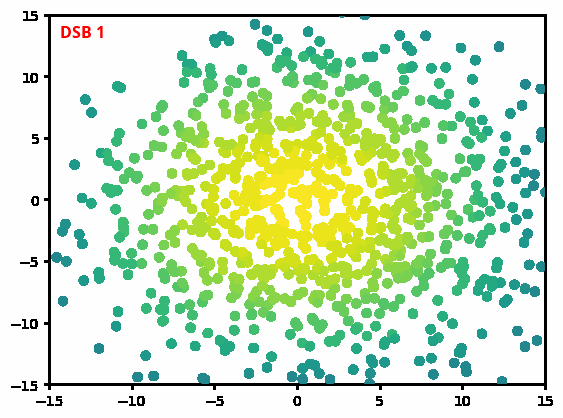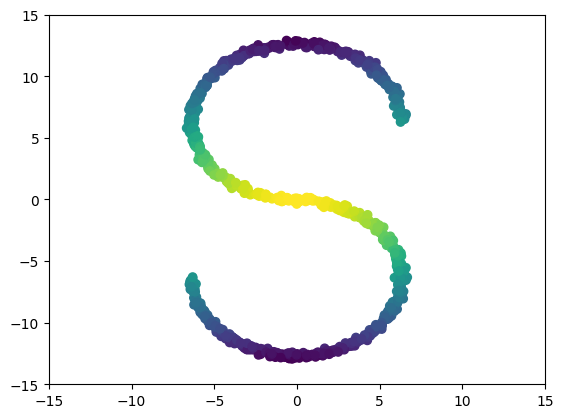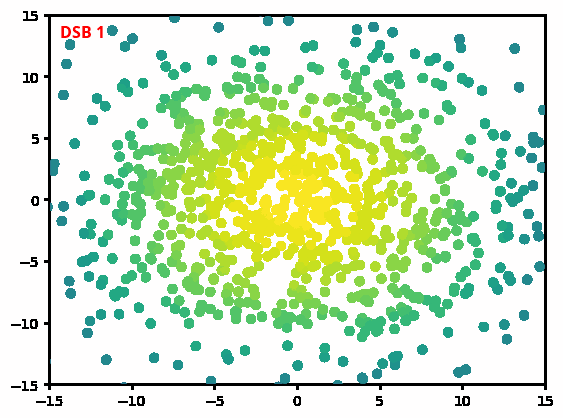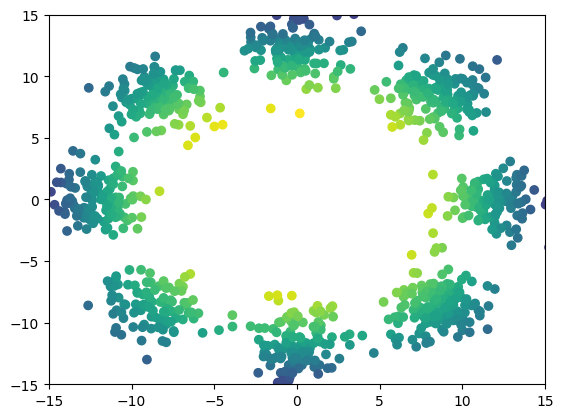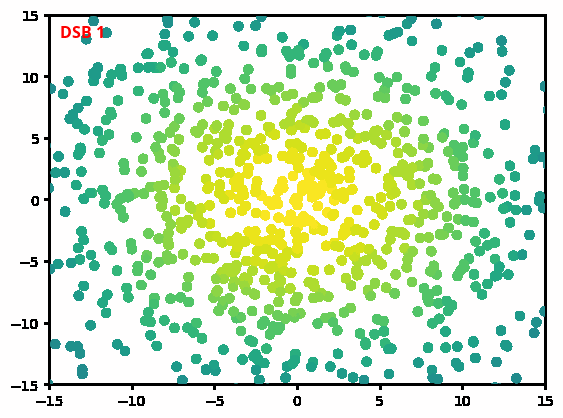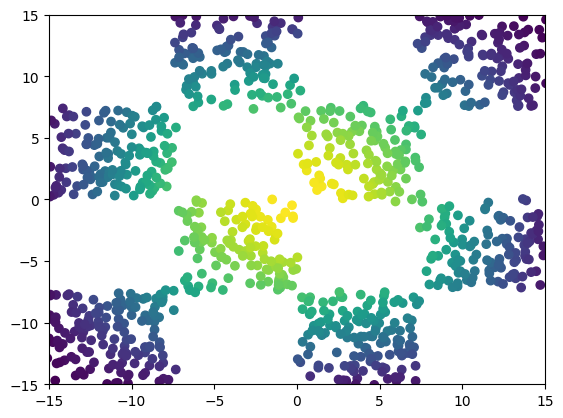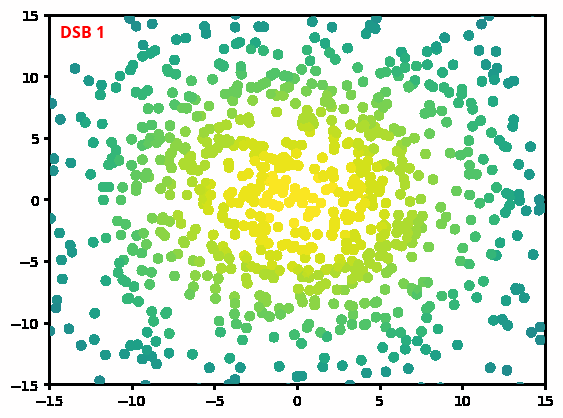## MNIST example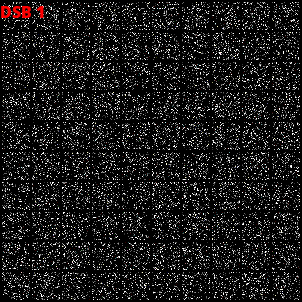## Dataset interpolation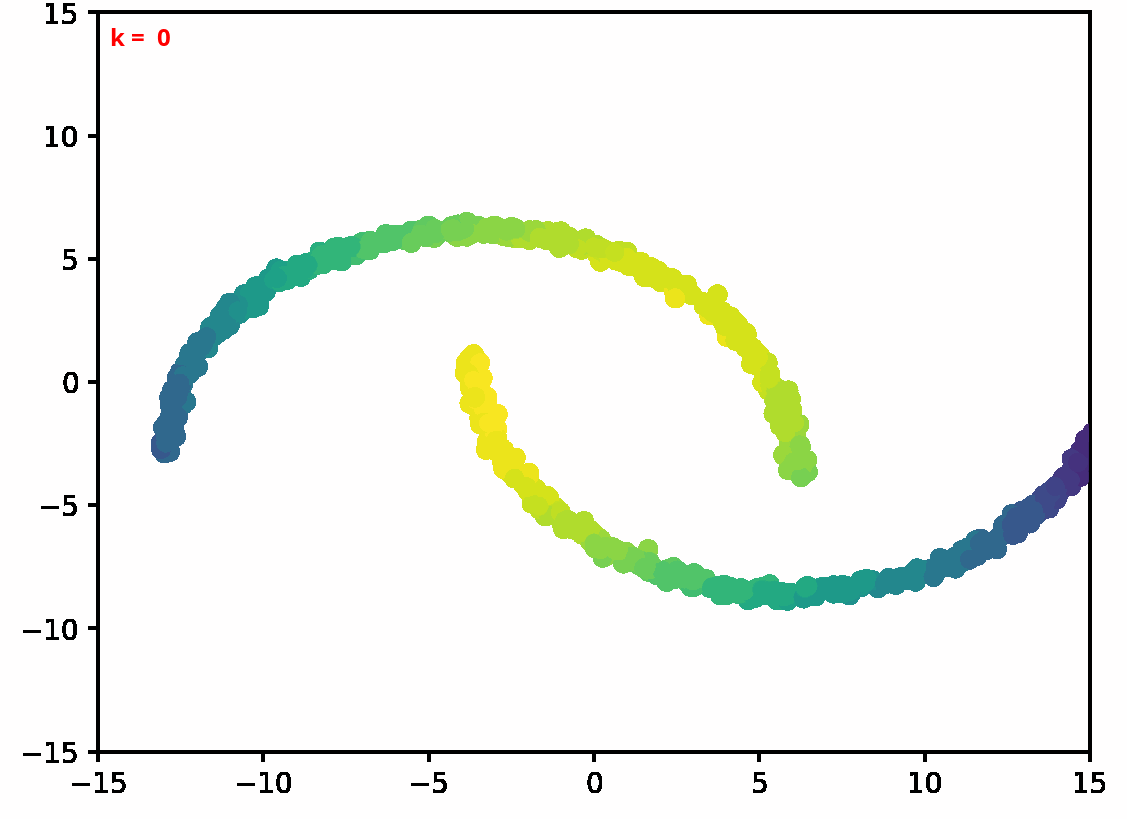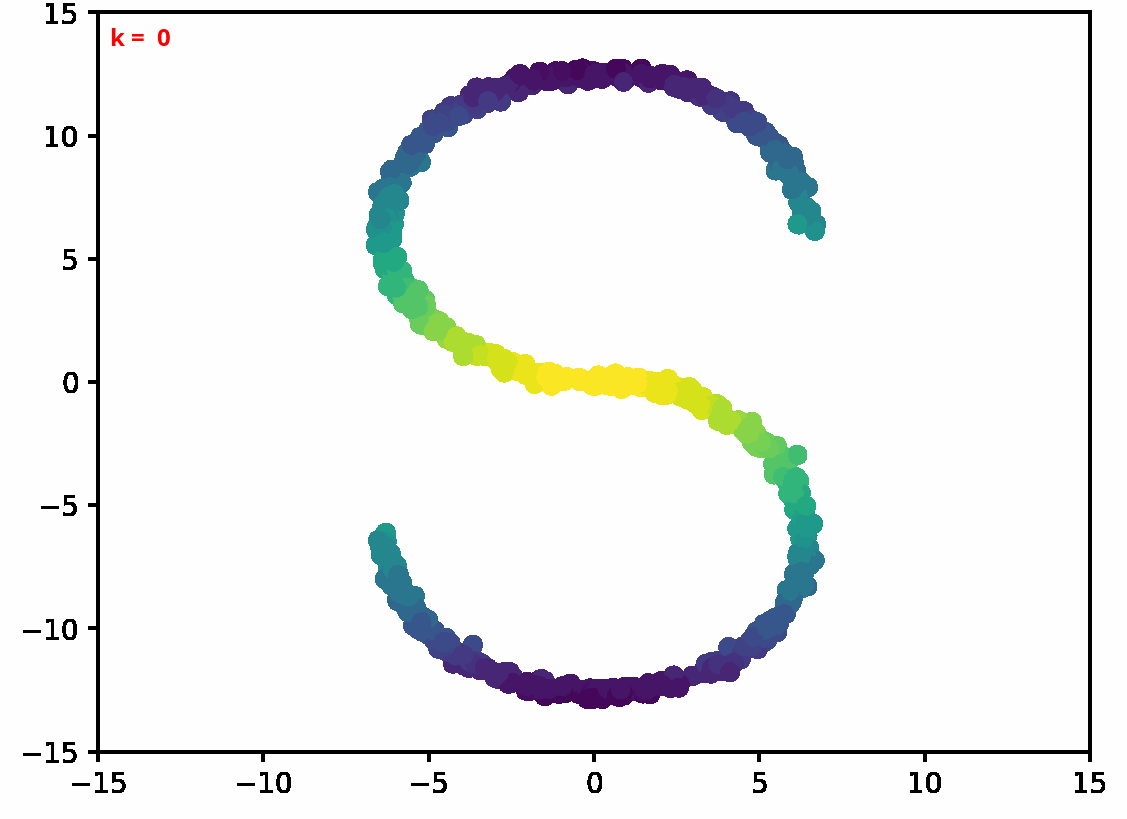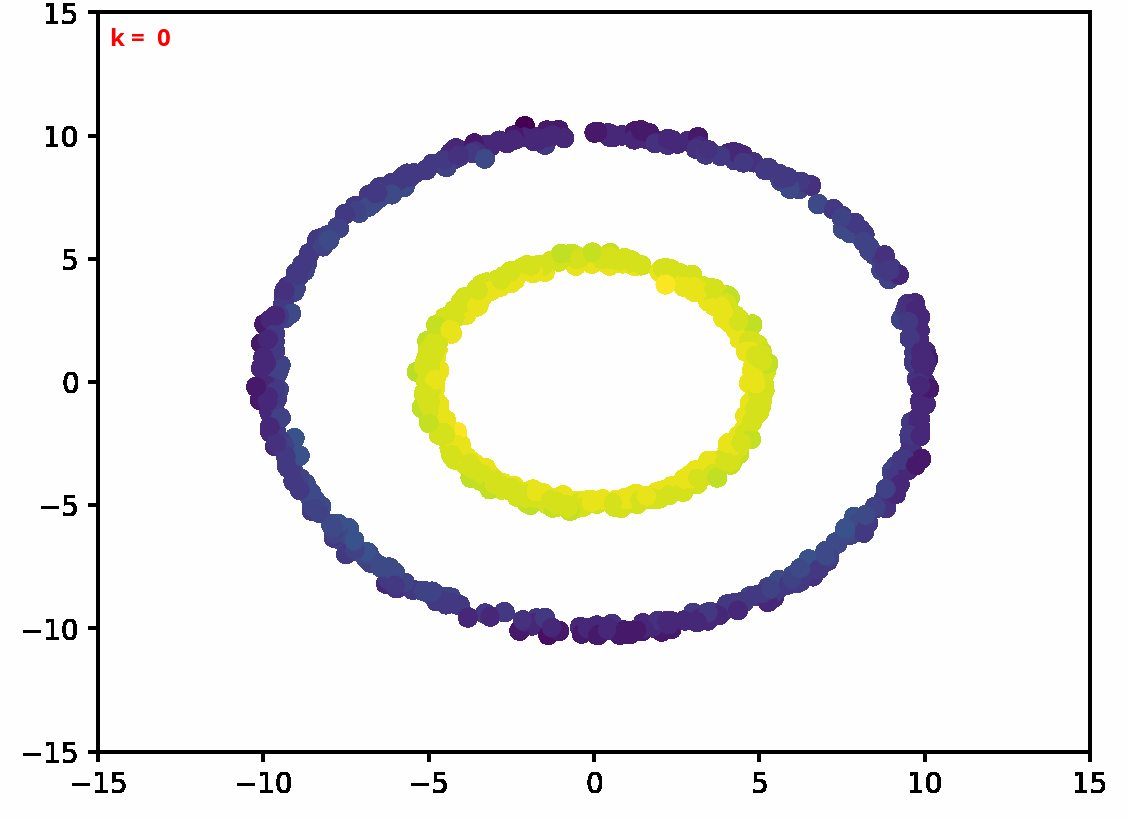Hans Föllmer Random fields and diffusion processes In: École d’été de Probabilités de Saint-Flour 1985-1987

 Christian Léonard A survey of the Schrödinger problem and some of its connections with optimal transport In: Discrete & Continuous Dynamical Systems-A 2014

 Yongxin Chen, Tryphon Georgiou and Michele Pavon Optimal Transport in Systems and Control In: Annual Review of Control, Robotics, and Autonomous Systems 2020

 Aapo Hyvärinen and Peter Dayan Estimation of non-normalized statistical models by score matching In: Journal of Machine Learning Research 2005

 Yang Song and Stefano Ermon Generative modeling by estimating gradients of the data distribution In: Advances in Neural Information Processing Systems 2019

 Jonathan Ho, Ajay Jain and Pieter Abbeel Denoising diffusion probabilistic models In: Advances in Neural Information Processing Systems 2020